# Binary Search in C

## Binary Search in C Language with Examples

In this article, we will see how we can perform Binary Search for searching an element in a given array in C Language with Examples. Please read our previous article where we discussed Linear Search in C Language with Examples. Let’s understand Binary Search in detail with a step-by-step explanation.

##### Binary Search:

For performing Binary Search, the condition is that the list of keys or elements should be sorted. Following is the sorted list with the size 15 and length also 15 (means the array is full).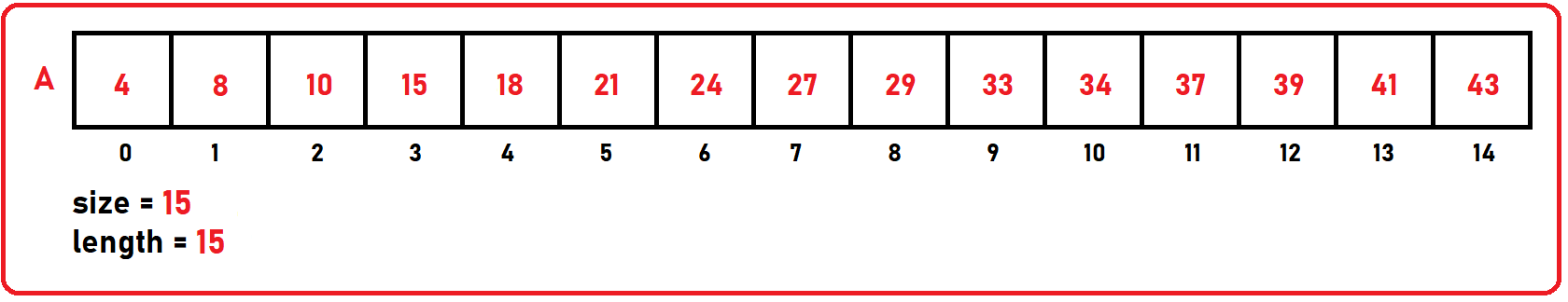Why we are calling this method a Binary Search? Because it will always check for the key element in the middle of the sorted list and it will split the list into two parts, so that’s why we call it Binary Search.

##### How Binary Search Works?

For example, Let’s take a key = 18, which is present at the index of 4 in the above array. If we perform a linear search, then how many comparisons will it do? 5 comparisons. For performing Binary Search, we need 3 index variables: – low, mid, and high. The value of mid will be calculated as: mid = (low + high) / 2.

Initially, low will point on the 0th index of the array and high will point on the last index of the array, in our case high will point on 14.

##### Example1 (key = 18):

Let us search element 18 in the above array. Following are the steps to search an element or key in a given array using Binary Search in C Language or any other language.

###### Step1: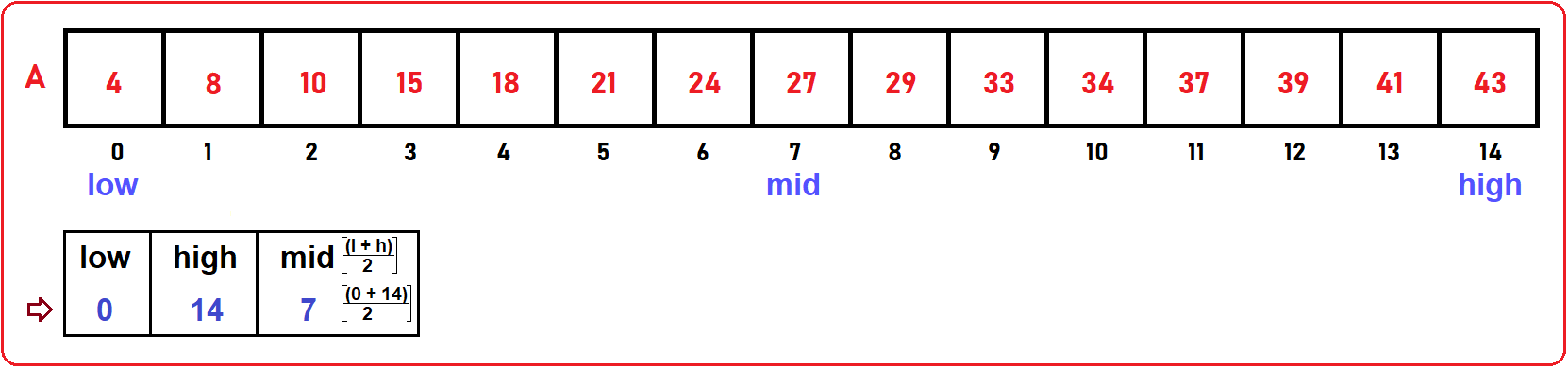We want to find the index of element 18. Our three variables will be initialized as: low: 0, high: 14, mid: 7

Check if (key == mid)? No, (18 < A ), then, check: If the key is smaller than mid, it will present on the left-hand side from the mid. Then modify high as high = mid – 1; If the key is larger than mid, it will present on the right-hand side from the mid. Then modify low as low = low + 1;

What we are doing here? We just separate our array into two parts and check where our key element can be found with the help of index variables.

###### Step2:

Check if (key == mid)? No, here key < mid (18 < A ), then we will modify variables as:

low = remains. i.e., 0

high = mid – 1; i.e., (7-1) = 6

mid = (low + high / 2); i.e., (0 + 6 / 2) = 3 which is shown in the below image.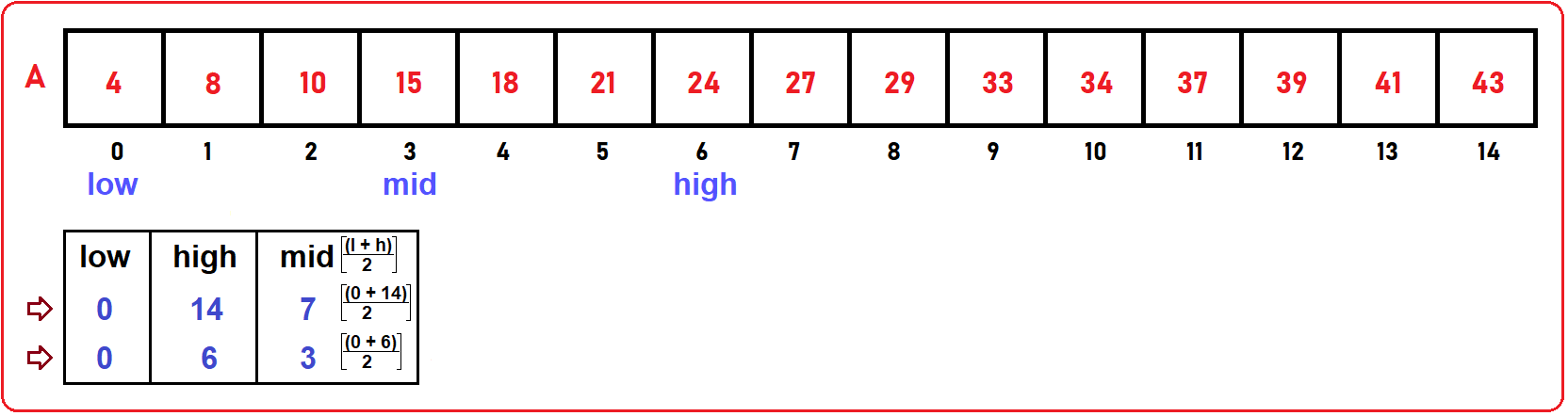###### Step3:

Check if (key == mid)? No, here key > mid (18 > A ), then we will modify variables as:

high = remains same as previous step. i.e., 6

low = mid + 1; i.e., (3 + 1) = 4

mid = (low + high / 2); i.e., (4 + 6 / 2) = 5 which is shown in the below image.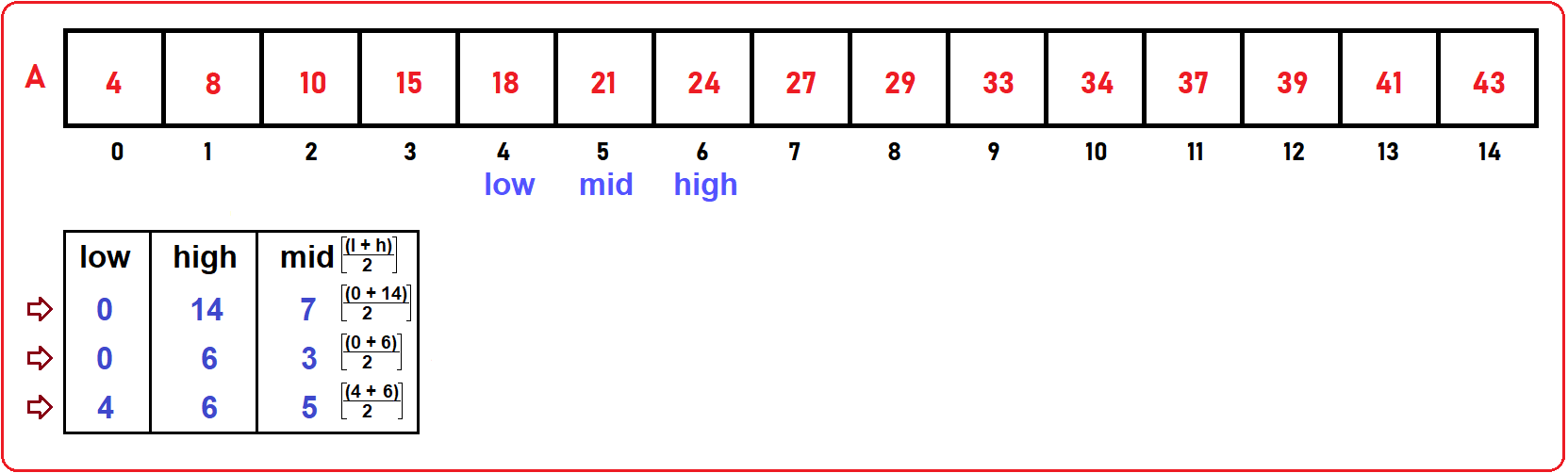###### Step4:

check if (key == mid)? No, here key > mid (18 < A ), then we will modify variables as:

low = remains same as previous step. i.e., 4

high = mid – 1; i.e., (5 – 1) = 4

mid = (low + high / 2); i.e., (4 + 4 / 2) = 4 which is shown in the below image.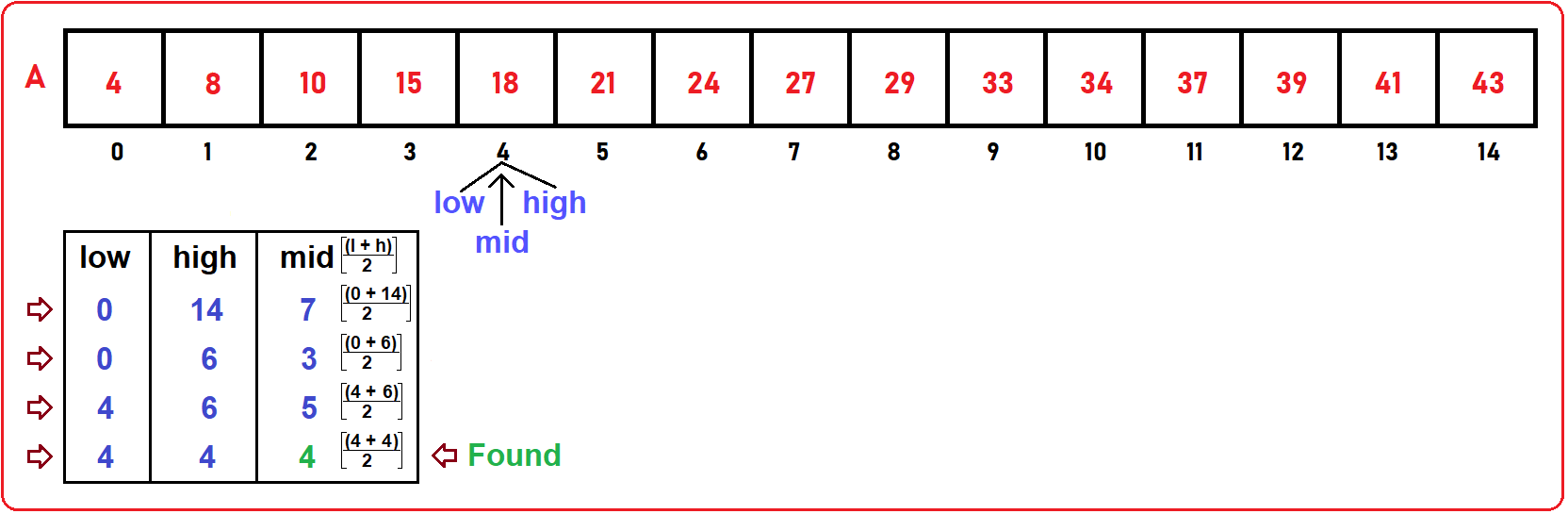Now check if (key == mid)? Yes, 18 == A , so in this way, we can perform the binary search. Here we take key element which was present in our array, now let’s take an example of the key element which is not present in the array:

##### Example2: (key = 25):
###### Step1:

We want to find the index of element 25. Our variable will be initialized as low: 0, high: 14, and mid: 7 as shown in the below image.###### Step2:

Check if (key == mid)? No, here key < mid (25 < A ), then we will modify variables as:

low = remains. i.e., 0

high = mid – 1; i.e., (7-1) = 6

mid = (low + high / 2); i.e., (0 + 6 / 2) = 3 which is shown in the below image.###### Step3:

Check if (key == mid)? No, here key > mid i.e. (25 > A ), then we need to modify variables as:

high = remains same as previous step. i.e., 6

low = mid + 1; i.e., (3 + 1) = 4

mid = (low + high / 2); i.e., (4 + 6 / 2) = 5 which is shown in the below image.###### Step4:

Check if (key == mid)? No, here key > mid (25 > A ), then we will modify variables as:

high = remains same as previous step. i.e., 6

low = mid + 1; i.e., (5 + 1) = 6

mid = (low + high / 2); i.e., (6 + 6 / 2) = 6 which is shown in the below image.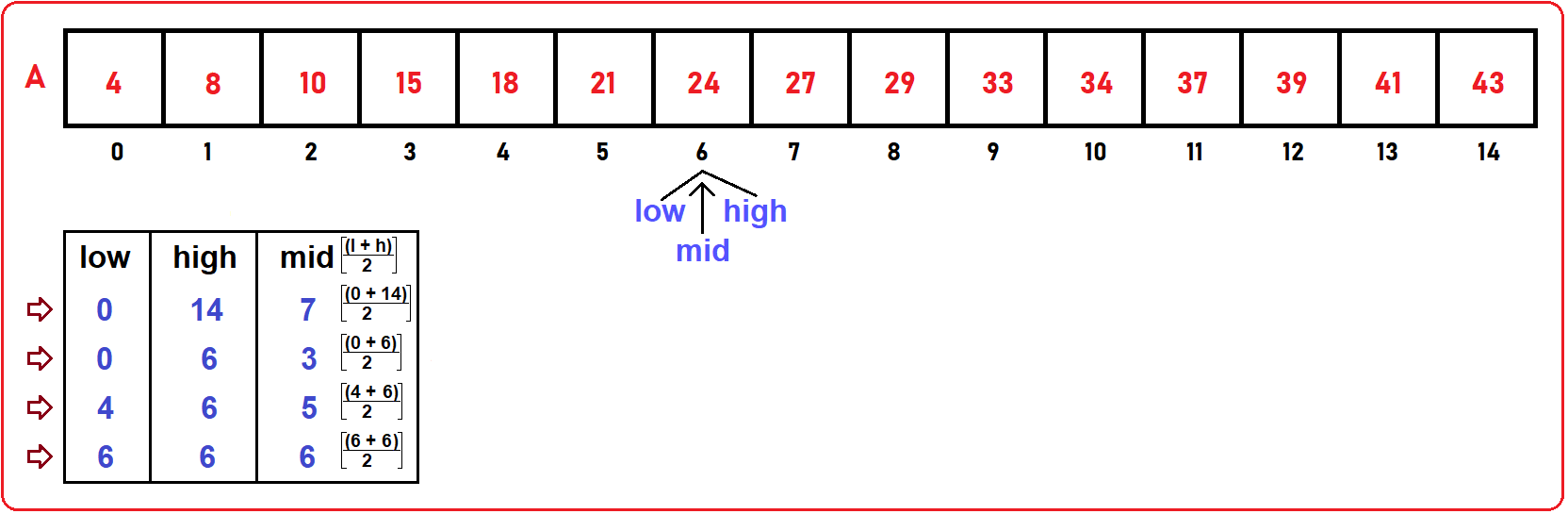###### Step5:

Check if (key == mid)? No, here key < mid (25 < A ), then we need to modify variables as:

low = remains same as previous step. i.e., 6

High = mid – 1; i.e., (6 – 1) = 5

mid = …

Here we will not calculate mid because high is less than low, here we have to break our condition and print key elements not found.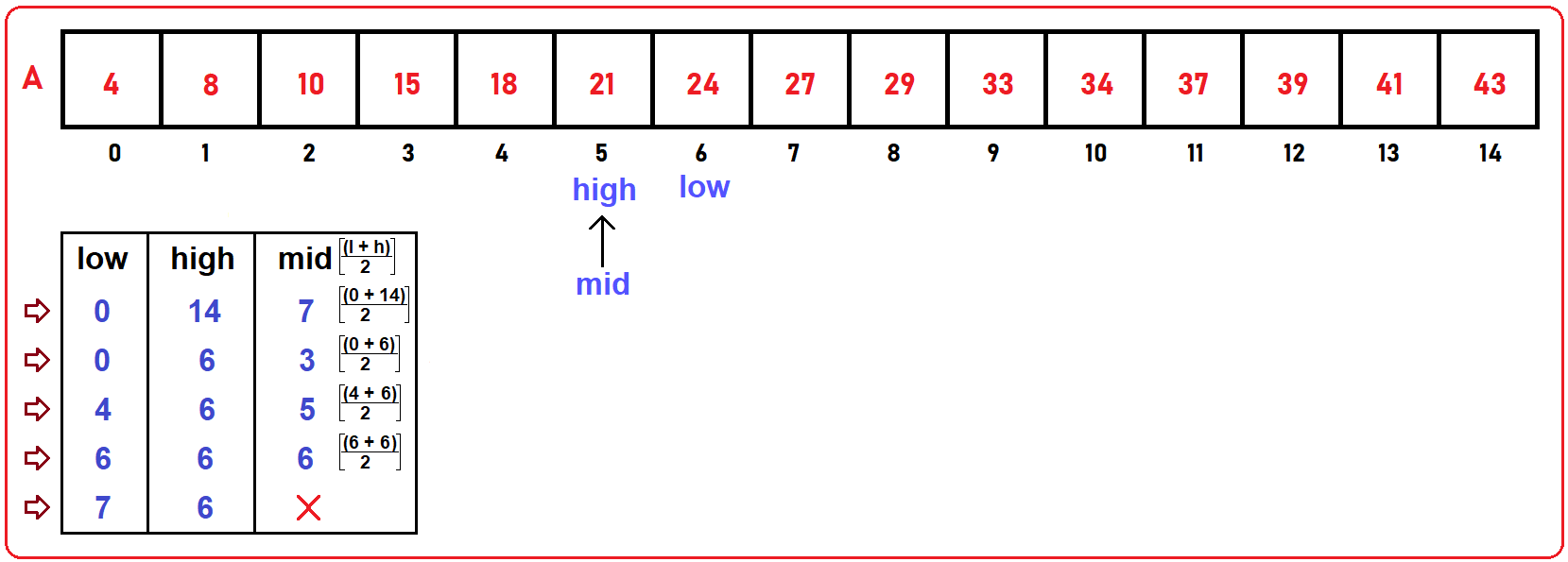As we have discussed both successful search and unsuccessful search, now let’s see how to implement this using C/C++ language.

##### Example Code of Binary Search:

As we saw in the above example, we have to calculate the mid variable in every step which will be modified by the low and high index variables. We have to remember that a low’s value should never be more than a high’s value. We can do the same task with loop and recursion also, let’s see both of them.

###### Iterative Version: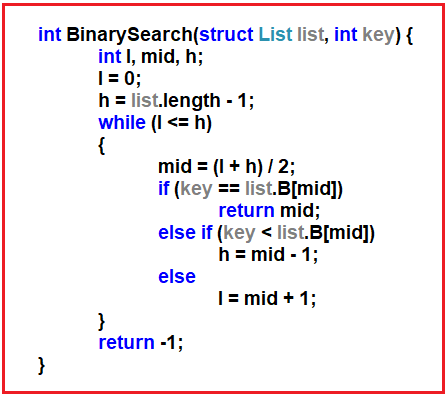###### Recursive Version: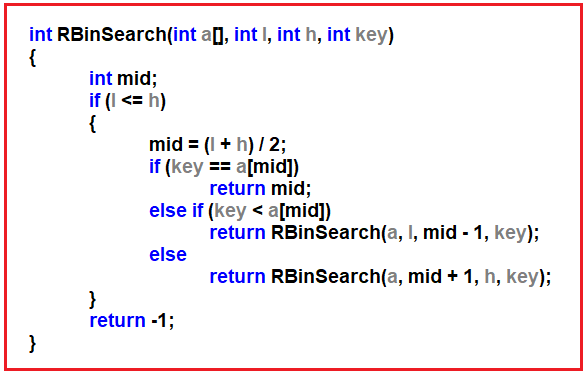##### Complete Binary Search Program in C Language using Recursion:

In the below program, we are using the Recursive approach to implement Binary Search to find an element in a given array using C language.

```#include <stdio.h>
#include <stdlib.h>
struct List
{
int size;
int length;
int B;
};

void Display (struct List list)
{
int i;
printf ("\nElements are\n");
for (i = 0; i < list.length; i++)
printf ("%d ", list.B[i]);
}

int RBinSearch (int a[], int l, int h, int key)
{

int mid;
if (l <= h)
{
mid = (l + h) / 2;
if (key == a[mid])
return mid;
else if (key < a[mid])
return RBinSearch (a, l, mid - 1, key);
else
return RBinSearch (a, mid + 1, h, key);
}
return -1;
}

int main ()
{
struct List list_1 = { 10, 8, {2, 3, 9, 16, 18, 21, 28, 32, 35} };
int x;

printf ("Enter the element which you want to search: \n");
scanf ("%d", &x);
printf ("Element present at index of %d\n", RBinSearch (list_1.B, 0, list_1.length, x));
}
```
###### Output: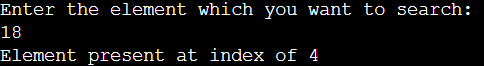##### Complete Binary Search Program in C Language using Iteration:

In the below program, we are using the Iterative approach to implement Binary Search to find an element in a given array using the C language.

```#include <stdio.h>
#include <stdlib.h>
struct List
{
int size;
int length;
int B;
};

void Display (struct List list)
{
int i;
printf ("\nElements are\n");
for (i = 0; i < list.length; i++)
printf ("%d ", list.B[i]);
}

int BinarySearch(struct List list, int key) {
int l, mid, h;
l = 0;
h = list.length - 1;
while (l <= h)
{
mid = (l + h) / 2;
if (key == list.B[mid])
return mid;
else if (key < list.B[mid])
h = mid - 1;
else
l = mid + 1;
}
return -1;
}

int main ()
{
struct List list_1 = { 10, 8, {2, 3, 9, 16, 18, 21, 28, 32, 35} };
int x;

printf ("Enter the element which you want to search: \n");
scanf ("%d", &x);
printf ("Element present at index of %d\n", BinarySearch(list_1, x));
}
```
###### Output:##### Binary Search Analysis:

We have seen how we can perform Binary Search to find an element in a given sorted array. Now, we will take a deep dive and do an Analysis of Binary Search. Let’s do the analysis.For the same list, we have searched for various keys, every time the middle value was 7 at the initial time. For example, if we want to find 27 then it will find by only one search.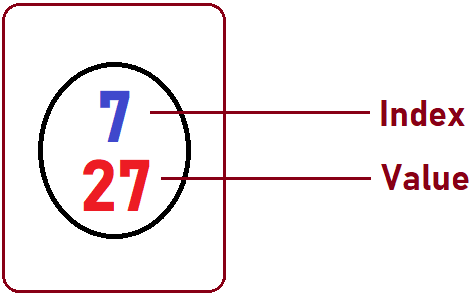It means the key value 27 at index 7will be found in just one single comparison. Let’s take another key which is 10. To search that, we will consider the left side of the array because 10 < 27. Now we want to find another key i.e., 39. Then we will consider the right side of the list i.e., 39 > 27. So here we divide the list into two parts.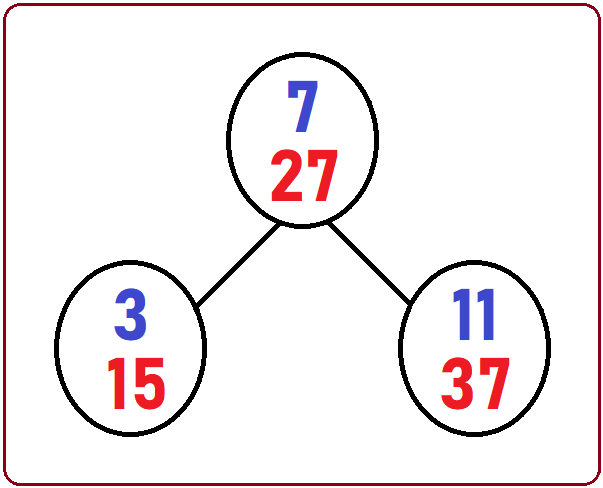If we are searching for targets 15 or 37 then these can be found in only 2 comparisons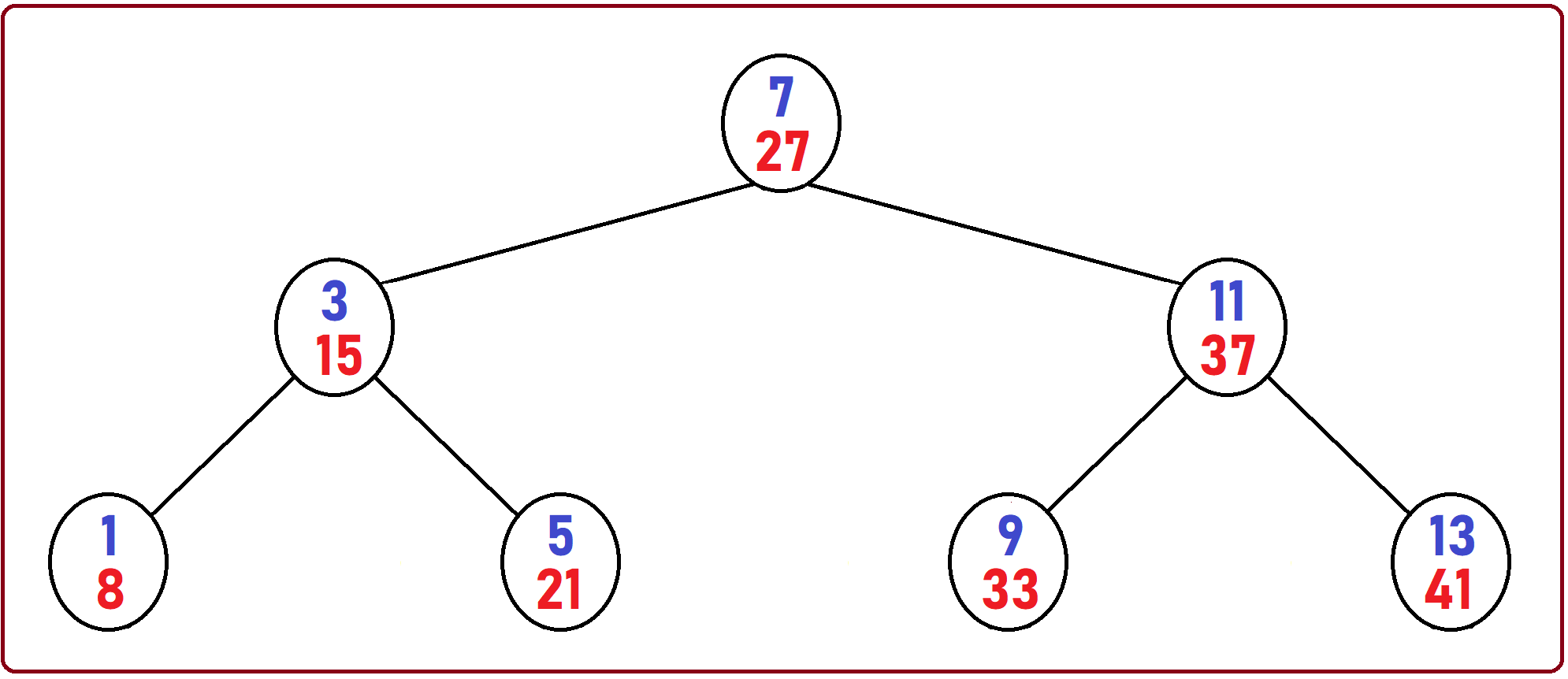If we are searching for targets 8, 21, 33, or 41 then these can be found in only 4 comparisons. If we compare this to linear search, the “41” element will be searched by linear search, then it takes 13 comparisons but if we do it with Binary Search then it will take only 4 comparisons.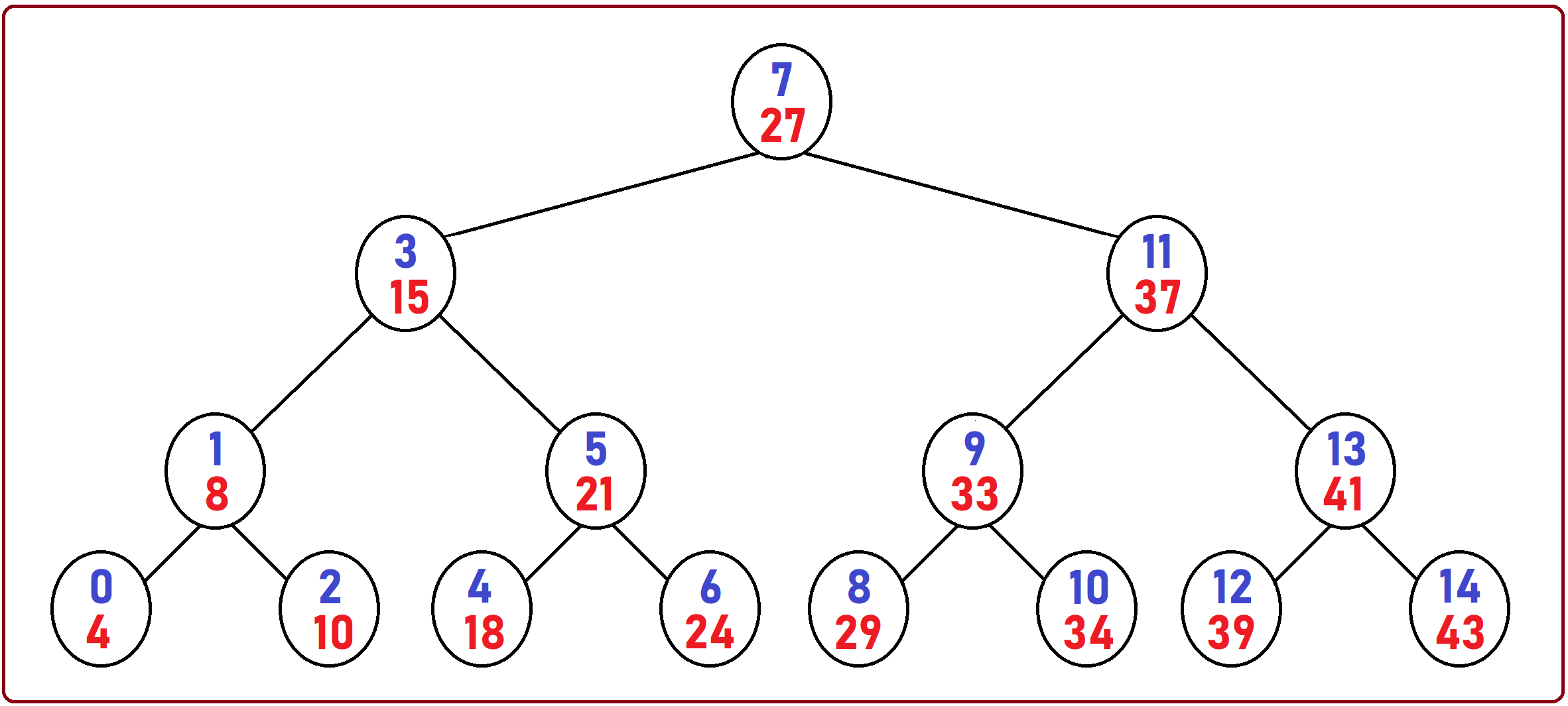Above is the full Comparison tree based on the Binary Search for our example Array which is used in this article.

27 — One Comparison

15, 37 — Two Comparisons

8, 21, 33, 41 — Three Comparisons

4, 10, 18, 25, 29, 34, 39, 43 — Four Comparisons

So Maximum how many comparisons are required – 4 Comparisons. Whether the element is present at the beginning of the list or at end of the list. So, the Number of comparisons depends on the height of the tree, which is logn,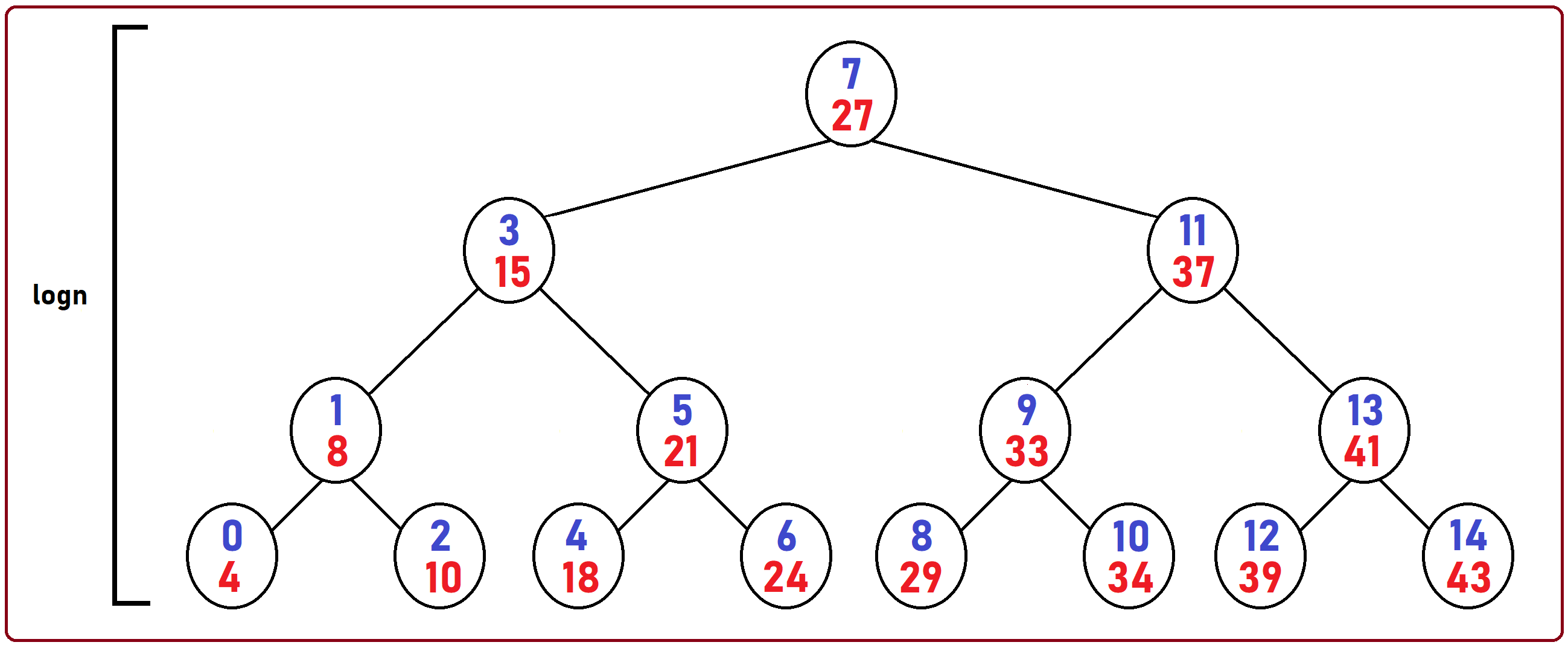The time taken will depend on the number of comparisons and comparisons are at most logn.

##### Time Complexity of Binary Search:
1. Best Case: O(1), when element found in only one comparison.
2. Worst Case: O(logn), when element found at last comparison, In our case 4th comparison.

For unsuccessful search, for example, if we pass 3 which is not present in our tree and it is smaller than 4, so all the comparisons will take place on the left-hand side as you can see on the above diagram, it will end to 0th index. Whether you write iterative or recursive process, time taken will always logn.

##### Average Case Analysis of Binary Search:

Now, we will see the Average Case Analysis of the Binary Search Algorithm. Let’s do the analysis. Let’s take an example of an array of size 15 and length 15:We have already seen the Binary Search comparison tree, now we want to analyze the average case in Binary Search.Above is the full comparison tree of Binary Search. Let’s take every row and derive the formula for average-case analysis.Here it will do 1 comparison so the equation will: 1 + …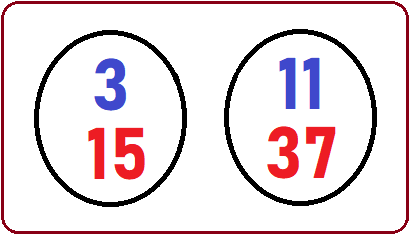Here again it will do 1 comparison so equation will: 1 + (1 * 2) + … (Number of comparisons * total number of elements)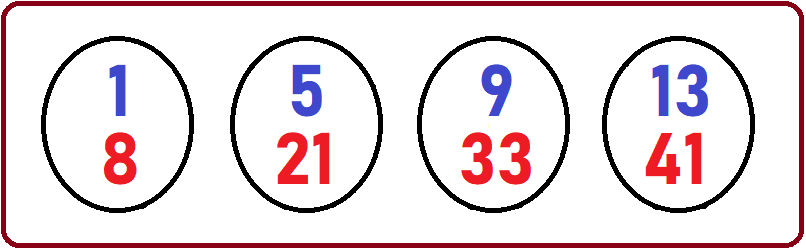Here again it will do 2 comparisons so equation will: 1 + (1 * 2) + (2 * 4) … (Number of comparisons * total number of elements)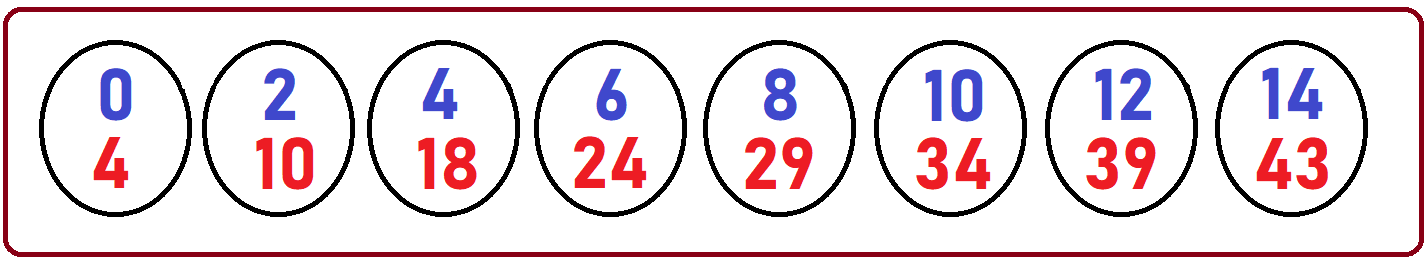Here again it will do 3 comparisons so equation will: 1 + (1 * 2) + (2 * 4) + (3 * 8) … (Number of comparisons * total number of elements)

Now, in the above-given array, there are 15 elements, but for the formula, we consider n elements. So, our equation will be: 1 + (1 * 2) + (2 * 4) + (3 * 8)

We can write the above equation as: 1 + (1 * 21) + (2 * 22) + (3 * 23)

We converted the number of elements to the powers of 2. Again, we can write the above equation as: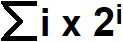So, in our case what is the range of i, it is i = 0 to i = logn.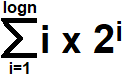Here logn is the height of the tree. Above represent the total time taken in all the possible cases. This is sum of the time taken in all possible cases as we have taken the total number of comparisons required for searching each and every element. Then this equation will be divided by n because total n elements are there. So, = Sum of all possible Cases/number of cases.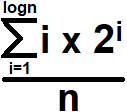What is the case here? Each element is the case present in the array. Now, the above equation can be written as: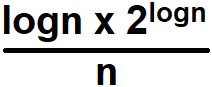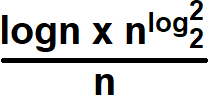We know the value of log2 2 is 1 and denominators and numerators will cancel each other so the answer will logn. The average case time taken is also logn.

As we have seen,

Best – O (1)

Worst – O (logn)

Average – O (logn)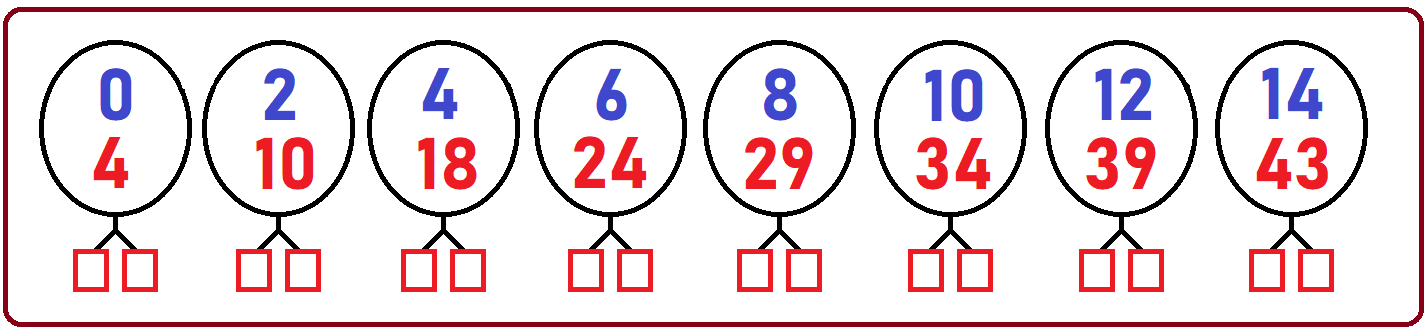In the above image, circular nodes are representing the successful search and below square nodes are representing the unsuccessful search. Let’s say these circular nodes as internal nodes and square nodes as External nodes.

I = It is the sum of the paths of all internal nodes.

E = It is the total of the paths of all external nodes.

Here is the relation between E and I,

E = I + 2n

The above equation will always be true. Let’s take an example of 2 paths of internal nodes, then there will be 8 external nodes.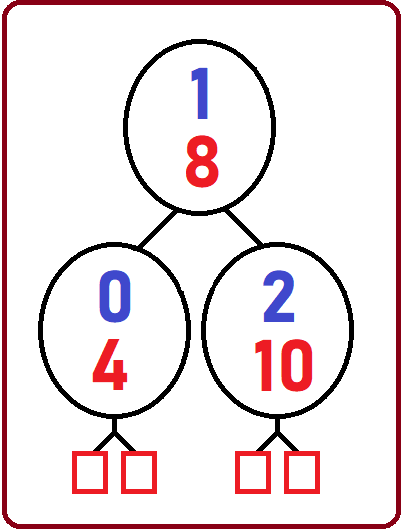In the above image, there are-

2 = sum of the path of internal nodes

8 = total number of external nodes

3 = n

Clearly, the above numbers satisfy the equation, E = I + 2n.

Average successful search time is:

A (n) = 1 + I/n

Here, in this article, I try to explain how we can perform Binary Search for searching an element in a given array in C Language with Examples and I hope you enjoy this Binary Search in C Language with Examples article.